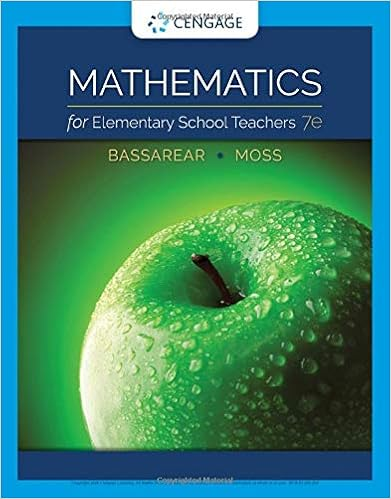Lucy baker is analyzing demographic characteristics

• Notes
• Mapache
• 30
• 96% (281) 271 out of 281 people found this document helpful

This preview shows page 8 - 11 out of 30 pages.

We have textbook solutions for you!
The document you are viewing contains questions related to this textbook.The document you are viewing contains questions related to this textbook.
Chapter 7 / Exercise 3
Mathematics for Elementary School Teachers
BassarearExpert Verified
26. Lucy Baker is analyzing demographic characteristics of two television programs, COPS (population 1) and 60 Minutes (population 2). Previous studies indicate no difference in the ages of the two audiences. (The mean age of each audience is the same.) Lucy plans to test this hypothesis using a random sample of 100 from each audience. Her null hypothesis is ____________. a) μ 1 - μ 2 b) μ 1 - μ 2 > c) μ 1 - μ 2 = 0 d) μ 1 - μ 2 < e) μ 1 - μ 2 < Ans: c 0 0 0 1 Response: See section 10.1 Hypothesis Testing and Confidence Intervals about the
Difference in Two Means using the z Statistic (Population Variances Known) Difficulty: Medium 27. Lucy Baker is analyzing demographic characteristics of two television programs, COPS (population 1) and 60 Minutes (population 2). Previous studies indicate no difference in the ages of the two audiences. (The mean age of each audience is the same.) Lucy plans to test this hypothesis using a random sample of 100 from each audience. Her alternate hypothesis is ____________.
We have textbook solutions for you!
The document you are viewing contains questions related to this textbook.The document you are viewing contains questions related to this textbook.
Chapter 7 / Exercise 3
Mathematics for Elementary School Teachers
BassarearExpert Verified
0 0 0 Response: See section 10.1 Hypothesis Testing and Confidence Intervals about the
Difference in Two Means using the z Statistic (Population Variances Known) Difficulty: Medium 28. Lucy Baker is analyzing demographic characteristics of two television programs, COPS (population 1) and 60 Minutes (population 2). Previous studies indicate no difference in the ages of the two audiences. (The mean age of each audience is the same.) Her staff randomly selected 100 people from each audience, and reported the following: x 1 = 43 years and x 2 = 45 years. Assume that σ 1 = 5 and σ 2 = 8. two-tail test and α = .05, the critical z values are _________________. With a Response: See section 10.1 Hypothesis Testing and Confidence Intervals about the
Difference in Two Means using the z Statistic (Population Variances Known) Difficulty: Medium 29. Lucy Baker is analyzing demographic characteristics of two television programs, COPS (population 1) and 60 Minutes (population 2). Previous studies indicate no difference in the ages of the two audiences. (The mean age of each audience is the same.) Her staff randomly selected 100 people from each audience, and reported the following: x 1 = 43 years and x 2 = 45 years. Assume that σ 1 = 5 and σ 2 = 8. Assuming a two-tail test and α = .05, the observed z value is _________________.
Response: See section 10.1 Hypothesis Testing and Confidence Intervals about the
Difference in Two Means using the z Statistic (Population Variances Known) Difficulty: Medium
•••